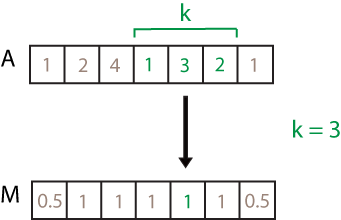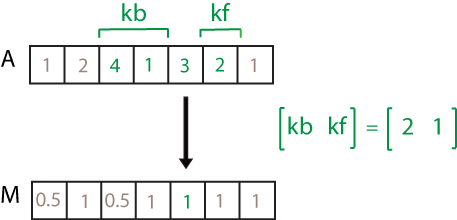## 语法

``M = movmad(A,k)``
``M = movmad(A,[kb kf])``
``M = movmad(___,dim)``
``M = movmad(___,nanflag)``
``M = movmad(___,Name,Value)``

## 说明

``M = movmad(A,k)` 返回由局部 `k` 个数据点的中位绝对偏差 (MAD) 组成的数组，其中每个 MAD 均基于 `A` 的相邻元素的长度为 `k` 的滑动窗计算得出。`M` 与 `A` 的大小相同。当 `k` 为奇数时，窗口以当前位置的元素为中心。当 `k` 为偶数时，窗口以当前元素及其前一个元素为中心。当没有足够的元素填满窗口时，窗口将自动在端点处截断。当窗口被截断时，只根据窗口内的元素计算 MAD。如果 `A` 是向量，`movmad` 将沿该向量的长度运算。如果 `A` 为多维数组，则 `movmad` 沿大小不等于 1 的第一个数组维度进行运算。`

``M = movmad(A,[kb kf])` 通过长度为 `kb+kf+1` 的窗口计算 MAD，其中包括当前位置的元素、后面的 `kb` 个元素和前面的 `kf` 个元素。`

``M = movmad(___,dim)` 为上述任意语法计算维度 `dim` 上的 MAD。例如，对于矩阵 `A`，`movmad(A,k,2)` 沿 `A` 的列进行运算，计算每行的 `k` 个元素的移动 MAD。`

``M = movmad(___,nanflag)` 指定在上述任意语法的计算中包括还是忽略 `NaN` 值。`movmad(A,k,'includenan')` 会在计算中包括所有 `NaN` 值，这是默认设置，而 `movmad(A,k,'omitnan')` 则忽略这些值并基于较少的点计算 MAD。`

``M = movmad(___,Name,Value)` 使用一个或多个名称-值对组参数指定移动 MAD 的其他参数。例如，如果 `x` 是时间值向量，则 `movmad(A,k,'SamplePoints',x)` 相对于 `x` 中的时间计算 `A` 的移动 MAD。`

## 示例

```A = [1 2 4 -1 -2 -3 -1 3 2 1]; M = movmad(A,3)```
```M = 1×10 0.5000 1.0000 2.0000 1.0000 1.0000 1.0000 2.0000 1.0000 1.0000 0.5000 ```

```A = [1 2 1 -1 -2 -3 -1 3 4 1]; M = movmad(A,[2 0])```
```M = 1×10 0 0.5000 0 1.0000 1.0000 1.0000 1.0000 2.0000 1.0000 1.0000 ```

`A = [1 2 1; -1 -2 -3; -1 3 4]`
```A = 3×3 1 2 1 -1 -2 -3 -1 3 4 ```
`M = movmad(A,3,2)`
```M = 3×3 0.5000 0 0.5000 0.5000 1.0000 0.5000 2.0000 1.0000 0.5000 ```

```A = [2 1 NaN -1 -2 -3 NaN 3 4 1]; M = movmad(A,3)```
```M = 1×10 0.5000 NaN NaN NaN 1.0000 NaN NaN NaN 1.0000 1.5000 ```

`M = movmad(A,3,'omitnan')`
```M = 1×10 0.5000 0.5000 1.0000 0.5000 1.0000 0.5000 3.0000 0.5000 1.0000 1.5000 ```

```A = [4 8 6 -1 -2 -3]; k = hours(3); t = datetime(2016,1,1,0,0,0) + hours(0:5)```
```t = 1x6 datetime Columns 1 through 3 01-Jan-2016 00:00:00 01-Jan-2016 01:00:00 01-Jan-2016 02:00:00 Columns 4 through 6 01-Jan-2016 03:00:00 01-Jan-2016 04:00:00 01-Jan-2016 05:00:00 ```
`M = movmad(A,k,'SamplePoints',t)`
```M = 1×6 2.0000 2.0000 2.0000 1.0000 1.0000 0.5000 ```

```A = [1 2 1 -1 -2 -3 -1 3 4 1]; M = movmad(A,3,'Endpoints','discard')```
```M = 1×8 0 1 1 1 1 2 1 1 ```

## 输入参数• 如果 `dim = 1`，则 `movmad(A,k,1)` 从第一列开始，垂直移过每行，每次计算 `k` 个元素的 MAD。然后移到第二列，重复上述计算。此过程持续到所有列都计算完为止。• 如果 `dim = 2`，则 `movmad(A,k,2)` 从第一行开始，水平移过每列，每次计算 `k` 个元素。然后移到第二行，重复上述计算。此过程持续到所有行都计算完为止。`NaN` 条件，指定为下列值之一：

• `'includenan'` - 计算 MAD 时包括输入中的 `NaN` 值，其对应输出为 `NaN` 值。

• `'omitnan'` - 忽略输入中的所有 `NaN` 值。如果窗口只包含 `NaN` 值，则 `movmad` 返回 `NaN`

### 名称-值对组参数

`'Endpoints'`说明
`'shrink'`在窗口接近输入端点时缩小窗口的大小，从而只包括现有元素。
`'discard'`当窗口不与现有元素完全重叠时，不输出任何 MAD 值。
`'fill'`将不存在的元素替换为 `NaN` 值。

## 详细信息

### 中位数绝对偏差

，其中 i = 1,2,...,N。